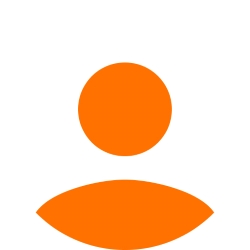inaivanova1990 31 Точки

## Heroes of Code 3

Здравейте, колеги! Може ли някой да ми помогне да разбера каква ми е грешката 80 / 100??

https://pastebin.com/DMU291W2

https://judge.softuni.org/Contests/Practice/Index/2303#2

Тагове:
0Axiomatik 2324 Точки

Problem was in the Heal + Recharge methods.

;-)

``````function solve(input) {
let num = +input.shift();
let index = 0;
let arrObjects = [];
let current = input.shift();

while (index < num) {
let obj = {};
let [hero, hitPoints, manaPoints] = current.split(' ');
hitPoints = +hitPoints;
manaPoints = +manaPoints;

obj = {
hero,
hitPoints,
manaPoints
};

arrObjects.push(obj)
current = input.shift();
index++;
}

while (current !== 'End') {
if (current.includes('CastSpell')) {
let [commandName, heroName, manaPoinstNeeded, spellName] = current.split(' - ');
arrObjects.filter(el => {
if (el.hero == heroName) {
let result = el.manaPoints - manaPoinstNeeded;

// if (el.manaPoints >= manaPoinstNeeded && el.manaPoints <= 200) {
if (el.manaPoints >= manaPoinstNeeded) {
el.manaPoints -= manaPoinstNeeded;
console.log(`\${el.hero} has successfully cast \${spellName} and now has \${result} MP!`)
} else {
console.log(`\${el.hero} does not have enough MP to cast \${spellName}!`)
}
}
});
} else if (current.includes('TakeDamage')) {
let [commandName, heroName, damage, attacker] = current.split(' - ');
arrObjects.filter(el => {
if (el.hero == heroName) {
damage = +damage;

if (el.hitPoints > damage) {
el.hitPoints -= damage;
console.log(`\${el.hero} was hit for \${damage} HP by \${attacker} and now has \${el.hitPoints} HP left!`)
} else {
console.log(`\${el.hero} has been killed by \${attacker}!`)
let index = arrObjects.indexOf(el);
arrObjects.splice(index, 1);
}
}
});
} else if (current.includes('Heal')) {
let [commandName, heroName, amount] = current.split(' - ');
amount = +amount;
arrObjects.filter(el => {
if (el.hero == heroName) {
let result = el.hitPoints + amount;
if (result <= 100) {
el.hitPoints += amount;
console.log(`\${el.hero} healed for \${amount} HP!`)
} else if (result > 100) {
// let residue = result - 100;
// amount = Math.abs(amount - residue);
let residue = 100 - el.hitPoints;
el.hitPoints = 100;
console.log(`\${el.hero} healed for \${residue} HP!`)
// console.log(`\${el.hero} healed for \${amount} HP!`)
}
}
})
} else if (current.includes('Recharge')) {
let [commandName, heroName, amount] = current.split(' - ');
amount = +amount;
arrObjects.filter(el => {
if (el.hero == heroName) {
let result = el.manaPoints + amount;

if (result > 200) {
// let residue = result - amount;
// amount = Math.abs(amount - residue);
let residue = 200 - el.manaPoints;
el.manaPoints = 200;
console.log(`\${el.hero} recharged for \${residue} MP!`)
} else {
el.manaPoints += amount;
console.log(`\${el.hero} recharged for \${amount} MP!`)
}
}
})
}

current = input.shift();
}

for (let line of arrObjects) {
console.log(`\${line.hero}
HP: \${line.hitPoints}
MP: \${line.manaPoints}`)
}
//console.log(arrObjects)

}``````

0inaivanova1990 31 Точки

Благодаря, мина всички тестове :)

1
23/07/2022 14:15:58
Можем ли да използваме бисквитки?
Ние използваме бисквитки и подобни технологии, за да предоставим нашите услуги. Можете да се съгласите с всички или част от тях.
Назад
Функционални
Използваме бисквитки и подобни технологии, за да предоставим нашите услуги. Използваме „сесийни“ бисквитки, за да Ви идентифицираме временно. Те се пазят само по време на активната употреба на услугите ни. След излизане от приложението, затваряне на браузъра или мобилното устройство, данните се трият. Използваме бисквитки, за да предоставим опцията „Запомни Ме“, която Ви позволява да използвате нашите услуги без да предоставяте потребителско име и парола. Допълнително е възможно да използваме бисквитки за да съхраняваме различни малки настройки, като избор на езика, позиции на менюта и персонализирано съдържание. Използваме бисквитки и за измерване на маркетинговите ни усилия.
Рекламни
Използваме бисквитки, за да измерваме маркетинг ефективността ни, броене на посещения, както и за проследяването дали дадено електронно писмо е било отворено.